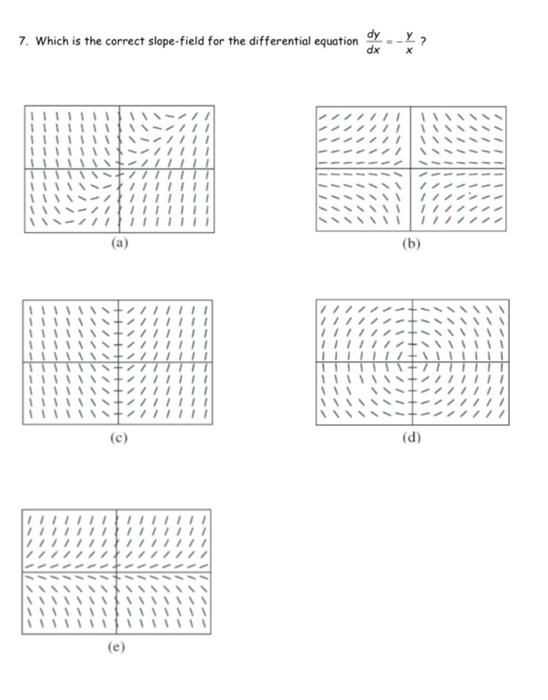### Create an Account

Home / Questions / 7. Which is the correct slope-field for the differential equation dy - 2 dx I // (b) (d) (e)

# 7. Which is the correct slope-field for the differential equation dy - 2 dx I // (b) (d) (e)

7. Which is the correct slope-field for the differential equation dy - 2 dx I // (b) (d) (e)May 15 2021 View more View LessSubscribe To Get Solution